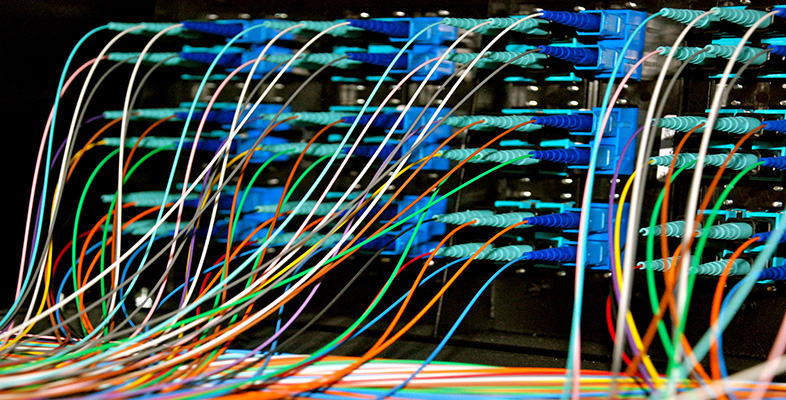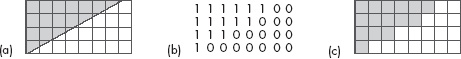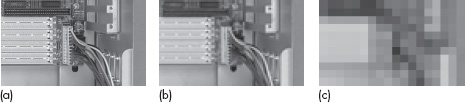Representing and manipulating data in computers

Start this free course now. Just create an account and sign in. Enrol and complete the course for a free statement of participation or digital badge if available.

Free course

# 3.2 Representing still images

There are two basic methods of representing still images in a computer: bit maps (also sometimes called raster graphics or raster images) and vector graphics (also sometimes called geometrical-shape graphics or graphics metafiles). Bit maps are usually used when there is a great deal of detail, as in photographs, or when there are irregular shapes, such as in drawings of natural objects. Vector graphics are usually reserved for line and blocked-colour drawings consisting of regular shapes. Examples of drawings for which it is appropriate to use vector graphics are flow charts, bar charts, engineering diagrams, etc.

## Activity 17 (Exploratory)

From the foregoing brief descriptions, which method of representing still images do you think that the digital camera will use?

### Discussion

It will use bit maps, because the images are photographs and so will have a great deal of detail.

Whether an image is obtained by taking a photograph with a digital camera or obtained by scanning a picture, the process of representing it digitally is similar. It involves dividing the image up into a grid of tiny squares called pixels (another name is ‘pels’ – both are abbreviations for ‘picture element’). Each pixel is then given a binary code representing its brightness and colour (or its shade of grey if it is a black and white image). This process is known as digitisation.

To give you an idea of the process, Figure 7 shows a bit-map representation for a very simple image – one that is purely black and white. Here each pixel can be represented by one bit: 1 for black and 0 for white, as in Figure 7(b). Notice, though, what happens where the grid cuts across the black/white boundary: if 50% or more of the square is black a 1 is used, otherwise a 0 is used. Clearly some of the detail of the original is lost in the digitisation process. When the image is reproduced, as in Figure 7(c), it will have jagged edges. This unwanted effect is sometimes called pixellation. Clearly, the smaller the pixels used the less the loss of detail will be apparent to the human eye and the closer the digital representation will seem to the original.Figure 7 A simple bit-map representation: (a) the original image with a grid of small squares superimposed; (b) the bit-map representation of the original image (1 represents black and 0 represents white); (c) the image which will be reproduced from the bit map

Figure 8(a) shows a black and white photograph reproduced as accurately as possible. Figure 8(b) shows how the photograph would look if it were digitised with a grid of 50 squares per inch. There is obviously some degradation of the image, but it is just about possible to see what the picture is. Figure 8(c) shows how it would look if it were digitised with a grid of just 10 squares per inch. You can clearly see the ‘blocky’ pixellation effect in this image – in fact the image is so pixellated that it is meaningless.Figure 8 (a) A black and white photograph of the interior of the casing for a PC, showing some of the sockets and cables; (b) the same photograph digitised at 50 squares per inch; (c) the same photograph digitised at 1 0 squares per inch

With colour images, if the brightness and colour change over the tiny square, as they very often will, then the binary code for that square will represent an average value for the pixel. This will again result in pixellation and hence some loss of detail when the image is reproduced. As with black and white images, the smaller the pixels used the closer the digital representation will seem to the original. Similarly, if only a few possible brightnesses and colours are used to represent each pixel then detail will again be lost and the digitised image will only approximate to the original. This time the remedy is to use more brightness values and colours. But increasing the number of pixels (by making each one smaller) means that more code words are needed to represent the image, and increasing the number of brightness values and colours means that each code word needs more bits. The result is that accurate bit-map representations of colour images (or even greyscale ones) need very large numbers of bits indeed and so form very large files. A compromise may have to be made between the file size and the closeness of the representation.

## Example 4

A colour picture which is 2 inches by 3 inches is to be bit-mapped at a density of 300 pixels per inch. (Pixel densities are conventionally described ‘per inch’ and so I have given the picture size in inches as well, to make the arithmetic easier.) A total of 256 brightness values and colours will be represented. How many bytes will this produce?

The 2-inch side will have 600 pixels and the 3-inch side 900. So there are 600 × 900 pixels in all, which is 540 000. 256 brightness values and colours will need 8 bits to represent them (remember: 256 is 28), which is 1 byte. Hence each pixel needs 1 byte and 540 000 bytes in total are needed. That's about half a million bytes – half a megabyte – for just one small picture!

In fact, 256 colour and brightness values aren't really enough to represent a full-colour image satisfactorily, and so in practice 3 bytes are often used to hold the brightness and colour. That increases the number of bytes for a small 2-inch by 3-inch picture to around one and a half million. As you can see, file sizes for images can be very large indeed, and that is why compression is frequently used, as I'll describe shortly.

## Activity 18 (Self assessment)

The digital camera introduced in this course produces images that are 2272 by 1712 pixels. Thirty bits are required to represent the colour and brightness data for each pixel. How much memory, in bytes, is required to store a single picture, assuming no compression?

An image of 2272 by 1712 pixels has a total of 3 889 664 pixels. If each of these requires 30 bits, the total number of bits needed to store one picture is 116 689 920, which is 14 586 240 bytes – over 14 megabytes! (In practice, 4 bytes may be used to hold each group of 30 bits, thus increasing the amount of space needed to store one picture to an even larger value.) This is why, in practice, digital cameras almost always carry out some compression before they store an image, so the image actually stored in the camera occupies less space.

## Box 6: Vector graphics

The alternative method of representing images, vector graphics, describes them by their shape. For example, a 2 cm square could be described as follows:

• Starting at the bottom left-hand corner, draw a 2 cm line vertically upwards.

• Draw a 2 cm line horizontally towards the right from the top of the previous line.

• Draw a 2 cm line vertically downwards from the end of the second line.

• Draw a 2 cm line from the end of the third line to the start of the first line.

By using an (x, y) co-ordinate system, this can be condensed to:

• start a new shape at (0,0)

• draw line to (0,2)

• draw line to (2,2)

• draw line to (2,0)

• draw line to start point.

Details such as the thickness and colour of the lines, whether the square is to be filled with colour and so on also need to be specified, but even so you can see that this representation is much more compact than the bit-map representation, and so will normally lead to smaller file sizes. But of course this representation does depend on the image being easy to break down into a number of standard shapes (rectangles, straight lines, simple curves, ovals, etc.).